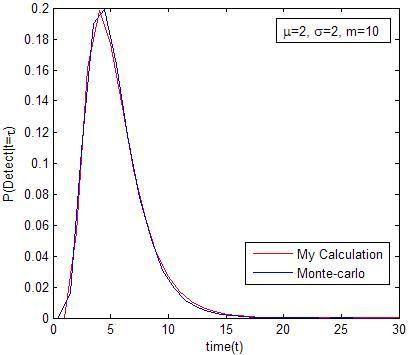top of page
Search
•Rana Basheer

# Hitting Time

Updated: Apr 2, 2021

This weekend I talked to my favorite professor about my previous supposedly simple probability problem. He quickly pointed out that this can be solved as a Hitting Time problem defined as

where

So Hitting Time is our detection time. The probability that this detection time is less than a given time t is given by

Since t is assumed to be continuous and at

Therefore,

However, if

So, the simplest way to make this discrete is to do the following simple approximation when

The figure below shows the result.Now that’s pretty close to monte-carlo simulations. Thanks Dr. Akim.

What did I learn. Sometimes discrete problems could be very difficult to mathematically solve (try computing the CDF of 25 random variables in my previous post). However, you can approximate the discrete problem by first computing the continuous time solution then using a simple discretization technique as above. I would like to find out the closed form solution when

5 views

See All
bottom of page